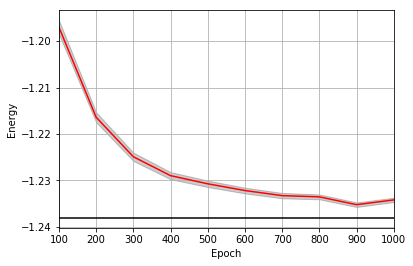This is a static, non-editable tutorial.

We recommend you install QuCumber if you want to run the examples locally. You can then get an archive file containing the examples from the relevant release here. Alternatively, you can launch an interactive online version, though it may be a bit slow:# Training while monitoring observables¶

As seen in the first tutorial that went through reconstructing the wavefunction describing the TFIM with 10 sites at its critical point, the user can evaluate the training in real time with the MetricEvaluator and custom functions. What is most likely more impactful in many cases is to calculate an observable, like the energy, during the training process. This is slightly more computationally involved than using the MetricEvaluator to evaluate functions because observables require that samples be drawn from the RBM.

Luckily, qucumber also has a module very similar to the MetricEvaluator, but for observables. This is called the ObservableEvaluator. The following implements the ObservableEvaluator to calculate the energy during the training on the TFIM data in the first tutorial. We will use the same hyperparameters as before.

It is assumed that the user has worked through tutorial 3 beforehand. Recall that quantum_ising_chain.py contains the TFIMChainEnergy class that inherits from the Observable module. The exact ground-state energy is -1.2381.

:

import os.path

import numpy as np
import matplotlib.pyplot as plt

from qucumber.nn_states import PositiveWaveFunction
from qucumber.callbacks import ObservableEvaluator

import qucumber.utils.data as data

from quantum_ising_chain import TFIMChainEnergy

:

train_data = data.load_data(
os.path.join("..", "Tutorial1_TrainPosRealWaveFunction", "tfim1d_data.txt")
)

nv = train_data.shape[-1]
nh = nv

nn_state = PositiveWaveFunction(num_visible=nv, num_hidden=nh)

epochs = 1000
pbs = 100  # pos_batch_size
nbs = 200  # neg_batch_size
lr = 0.01
k = 10

log_every = 100

h = 1
num_samples = 10000
burn_in = 100
steps = 100

tfim_energy = TFIMChainEnergy(h)


Now, the ObservableEvaluator can be called. The ObservableEvaluator requires the following arguments.

1. log_every: the frequency of the training evaluators being calculated is controlled by the log_every argument (e.g. log_every = 200 means that the MetricEvaluator will update the user every 200 epochs)
2. A list of Observable objects you would like to reference to evaluate the training (arguments required for generating samples to calculate the observables are keyword arguments placed after the list)

The following additional arguments are needed to calculate the statistics on the generated samples during training (these are the arguments of the statistics function in the Observable module, minus the nn_state argument; this gets passed in as an argument to fit).

• num_samples: the number of samples to generate internally
• num_chains: the number of Markov chains to run in parallel (default = 0)
• burn_in: the number of Gibbs steps to perform before recording any samples (default = 1000)
• steps: the number of Gibbs steps to perform between each sample (default = 1)

The training evaluators can be printed out via the verbose=True statement.

:

callbacks = [
ObservableEvaluator(
log_every,
[tfim_energy],
verbose=True,
num_samples=num_samples,
burn_in=burn_in,
steps=steps,
)
]

nn_state.fit(
train_data,
epochs=epochs,
pos_batch_size=pbs,
neg_batch_size=nbs,
lr=lr,
k=k,
callbacks=callbacks,
)

Epoch: 100
TFIMChainEnergy:
mean: -1.197008     variance: 0.022882      std_error: 0.001513
Epoch: 200
TFIMChainEnergy:
mean: -1.216405     variance: 0.011845      std_error: 0.001088
Epoch: 300
TFIMChainEnergy:
mean: -1.224917     variance: 0.008271      std_error: 0.000909
Epoch: 400
TFIMChainEnergy:
mean: -1.228988     variance: 0.005977      std_error: 0.000773
Epoch: 500
TFIMChainEnergy:
mean: -1.230748     variance: 0.004908      std_error: 0.000701
Epoch: 600
TFIMChainEnergy:
mean: -1.232201     variance: 0.003850      std_error: 0.000620
Epoch: 700
TFIMChainEnergy:
mean: -1.233295     variance: 0.003404      std_error: 0.000583
Epoch: 800
TFIMChainEnergy:
mean: -1.233561     variance: 0.002842      std_error: 0.000533
Epoch: 900
TFIMChainEnergy:
mean: -1.235249     variance: 0.002480      std_error: 0.000498
Epoch: 1000
TFIMChainEnergy:
mean: -1.234220     variance: 0.002232      std_error: 0.000472


The callbacks list returns a list of dictionaries. The mean, standard error and the variance at each epoch can be accessed as follows.

:

energies = callbacks.TFIMChainEnergy.mean
errors = callbacks.TFIMChainEnergy.std_error
variance = callbacks.TFIMChainEnergy.variance
# Please note that the name of the observable class that the user makes must be what comes after callbacks.


A plot of the energy as a function of the training cycle is presented below.

:

epoch = np.arange(log_every, epochs + 1, log_every)

E0 = -1.2381

ax = plt.axes()
ax.plot(epoch, energies, color="red")
ax.set_xlim(log_every, epochs)
ax.axhline(E0, color="black")
ax.fill_between(epoch, energies - errors, energies + errors, alpha=0.2, color="black")
ax.set_xlabel("Epoch")
ax.set_ylabel("Energy")
ax.grid()